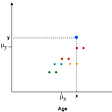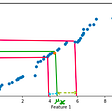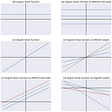# Probability theory: Bayes’ theorem

This is part of the course “Probability Theory and Statistics for Programmers”.

Bayes’ theorem looks like this:

Where A and B are events.

P(A|B) —the likelihood of event A occurring after B is tested

P(B|A) — the likelihood of event B occurring after A is tested

P(A) and P(B) — probabilities of events A and B

One of the most popular examples is calculating the probability of having a rear disease. Let’s imagine that some person comes back from an exotic country and want to check, does he have this country rear disease. He took a test and got 0.9 that he has a disease, given that the test has 0.99 probability of giving the right result. Also, this disease is very unlikely to occur, since only 0.0001 people returning from the country have the disease.

P(A|B) — the probability that a person has a disease—?

P(B|A) — the probability that person has a disease(after he took a test)— 0.9

P(A) — the probability that a person can have a disease — 0.0001

P(B) —the probability that the test gave a true result — ?

Let’s calculate P(B) with “The Law Of Total Probability”:

Now we can calculate P(A|B):

After calculating real probability we can see that real probability quite differs from probability, given by disease test. Now, in order to find a better probability, the person can go to other disease test providers and try to find a more precise probability. Because now he will use 0.0009 rather than 0.0001 as P(A).

Next part ->

Reach the next level of focus and productivity with increaser.org.

--

--

--

Indie hacker behind increaser.org. More at radzion.com

Love podcasts or audiobooks? Learn on the go with our new app.

## Scientific Computation using Matlab: part2## Covariance and Correlation## Miracles of number 1.618 (Part 1)## Markov Chain Analysis and Simulation using Python## A Guide to Metrics in Exploratory Data Analysis## Euler Totient Function in its core!## Probability theory: Moments, Variance, Standard deviation## Probability theory: Basic conceptsIndie hacker behind increaser.org. More at radzion.com

## What are Descriptive Statistics?## Understanding maths behind covariance and implementation using Numpy## Linear Functions